/

### The simplest factor structures for a risk model

The simplest sort of factor-based risk model is one that has an underlying multivariate normal distribution. Its structure then derives from the covariance matrix underlying the multivariate normal distribution.

The simplest factor structure for such a risk model involvingdifferent factors has a covariance matrix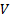which has the form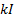, whereis the identity matrix.

The next simplest factor structure is arguably one where the covariance matrix is a diagonal matrix (i.e. the elements of, i.e.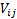are non-zero only when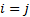. Its correlation matrix is therefore still the identity matrix.

The next simplest factor structure is arguably one where the the correlation matrix,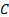, has elements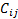where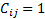ifand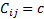if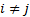whereis the same for all such values ofand.